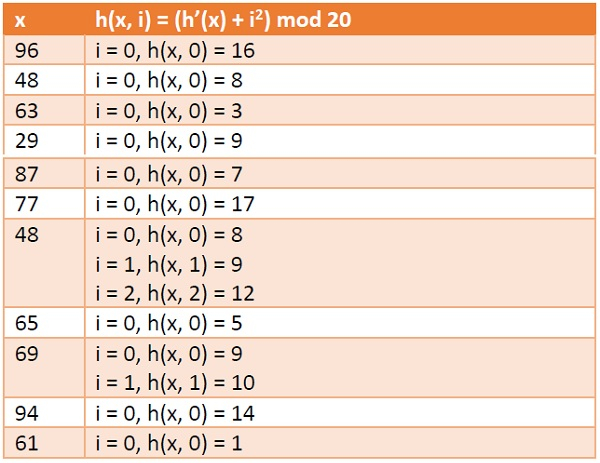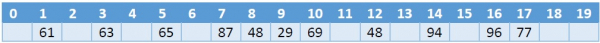# Double Hashing in Data Structure

In this section we will see what is Double Hashing technique in open addressing scheme. There is an ordinary hash function h´(x) : U → {0, 1, . . ., m – 1}. In open addressing scheme, the actual hash function h(x) is taking the ordinary hash function h’(x) when the space is not empty, then perform another hash function to get some space to insert.

$$h_{1}(x)=x\:mod\:m$$

$$h_{2}(x)=x\:mod\:m^{\prime}$$

$$h(x,i)=(h^{1}(x)+ih^{2})\:mod\:m$$

The value of i = 0, 1, . . ., m – 1. So we start from i = 0, and increase this until we get one free space. So initially when i = 0, then the h(x, i) is same as h´(x).

## Example

Suppose we have a list of size 20 (m = 20). We want to put some elements in linear probing fashion. The elements are {96, 48, 63, 29, 87, 77, 48, 65, 69, 94, 61}

$$h_{1}(x)=x\:mod\:20$$

$$h_{2}(x)=x\:mod\:13$$

x h(x, i) = (h1 (x) + ih2(x)) mod 20Hash Table